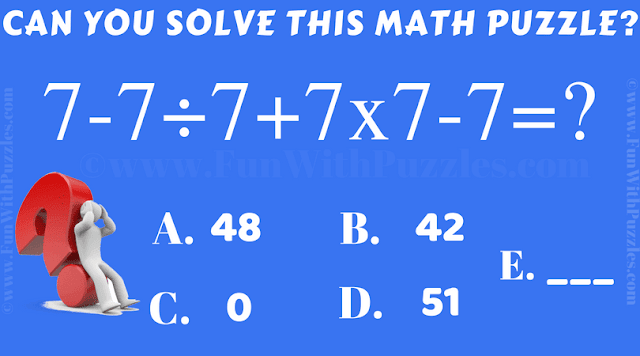This Math Riddle Brain Teaser is for Kids who has learned basics of Mathematics. Solving this Maths Equation Brain Teaser will require the basic BODMAS knowledge of Mathematics. This Math Brain Teaser is for Kids, but many teens and adults may calculate the value of given number equation wrongly if the basic concepts of Mathematics are not clear. Lets see if you can solve this Math Riddle Brain Teaser correct in your first attempt?Can you solve this Math Riddle Brain Teaser?

Answer of this "Math Riddle Brain Teaser for Kids", can be viewed by clicking on answer button. Please do give your best try before looking at the answer.

Ketill said...

I'm sorry, but the answer is NOT A. the answer is -50. Please explain how you get 48 as the answer.

Rajesh Kumar said...

Please apply the BODMAS to get the answer to this mathematical puzzle. Please put the details how you could get answer as -50?

Jimmy said...

It is 0